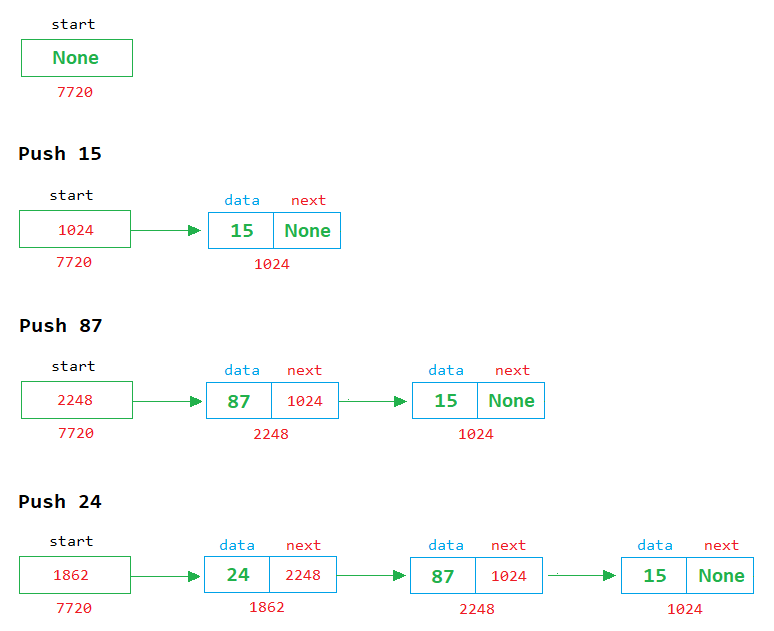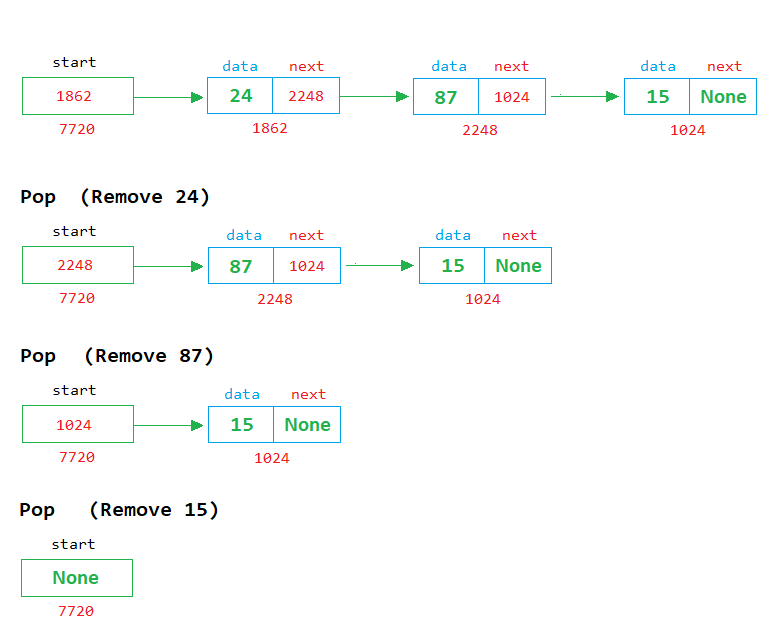# Stack using Linked List in Python

###### User-Defined Data Structures in Python

In this lesson, we will learn how to implement the stack using a singly linked list in python.

## Linked List Implementation of Stack in Python

We know about the stack and how to implement it using an array. In this lesson, we will learn how to implement the stack using a singly linked list.

We also know that two operations on the stack are possible: push and pop. See the image below to understand how to implement push and pop operations on a stack using a linked list.

### Push ExampleIn the above image, we can see that when a new node is created, it is placed in front of the first node, and its address is stored in the start variable.

### Pop ExampleIn the above image, we can see that each time a node is removed, the first node is deleted from the list, and the address of the next node is stored in the start variable.## Program of Stack using Linked List

Below is the complete program of stack in python using singly linked list having size 5.

#### Example

``````import os

# define node class
class Node:
def __init__(self):
self.data = 0
self.next = None

start = None
temp = None
size = 5
top = 0
ch = None
n = None

while 1:
os.system('cls')
print('1. Push')
print('2. Pop')
print('3. Display')
print('4. Exit')

if ch==1:   # for push operation

if top==size:
print('Stack is full')
input('Press enter to continue...')     # Pause the loop so that the user can see the message

else:
# Enter a number to store in node
n=int(input('Enter a number '))

# create a node object in temp
temp = Node()
temp.data = n           # assign the value of n in the data part of temp
temp.next = None        # assign the None in the next part of temp

if start == None:       # if start in None
start = temp        # then assing the temp in start (start points to the first node in the memory)

else:
# insert the new node before the first node
temp.next=start;
start=temp;
top=top+1

elif ch==2:     # for pop operation

if start==None:
print('Stack is empty')
input('Press enter to continue...')     # Pause the loop so that the user can see the message

else:
print('Number Popped = %d' %(start.data))
temp=start
start=start.next
del(temp)
top=top-1
input('Press enter to continue...')     # Pause the loop so that the user can see the message

elif ch==3:     # for display operation

if start==None:
print('Stack is empty')
input('Press enter to continue...')     # Pause the loop so that the user can see the message

else:
temp=start		# start from 1st node
# display the nodes on the screen
while temp is not None:
print('%d' %(temp.data))
temp=temp.next
input('Press enter to continue...')     # Pause the loop so that the user can see the stack

elif ch==4:
exit(0)

else:
print('Wrong Choice')
input('Press enter to continue...')     # Pause the loop so that the user can see the message``````